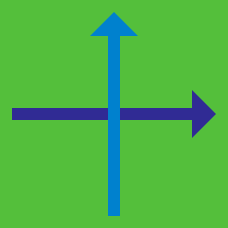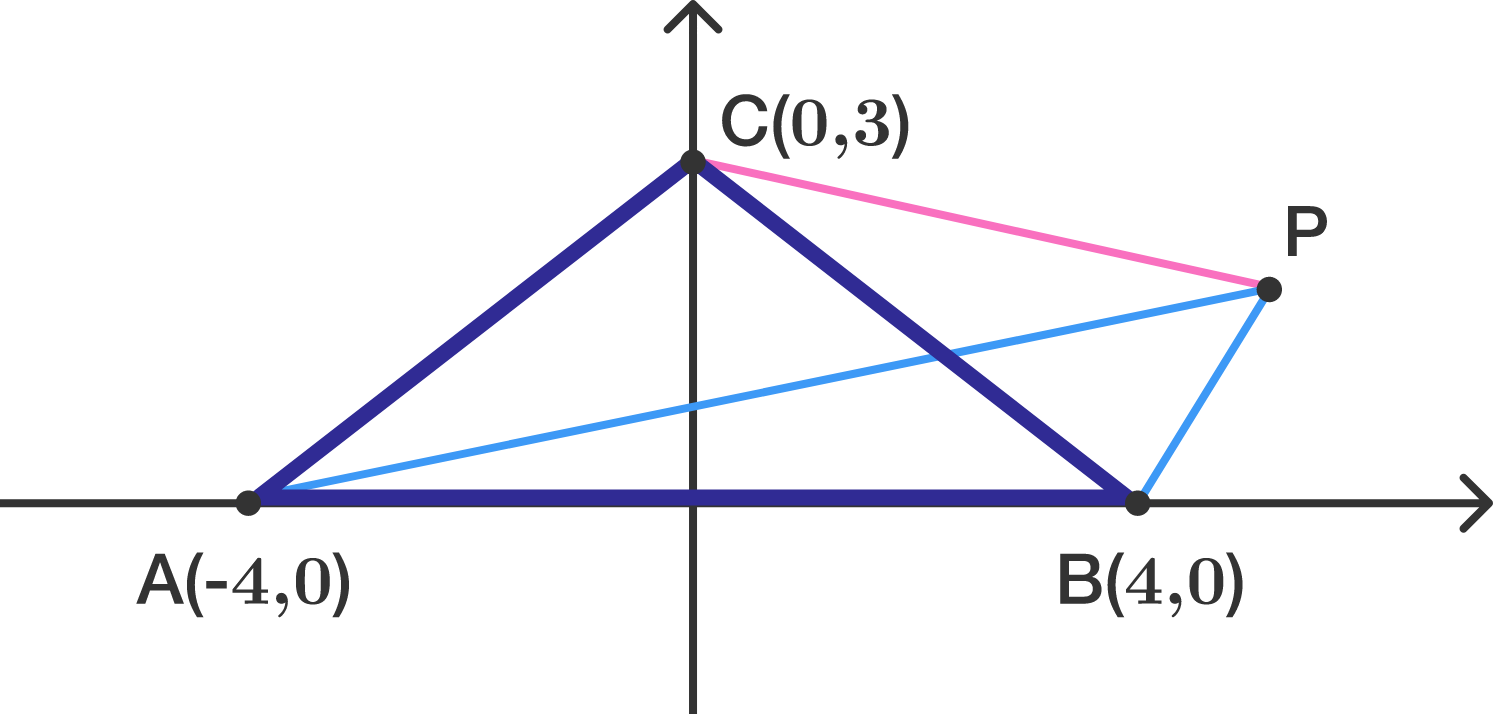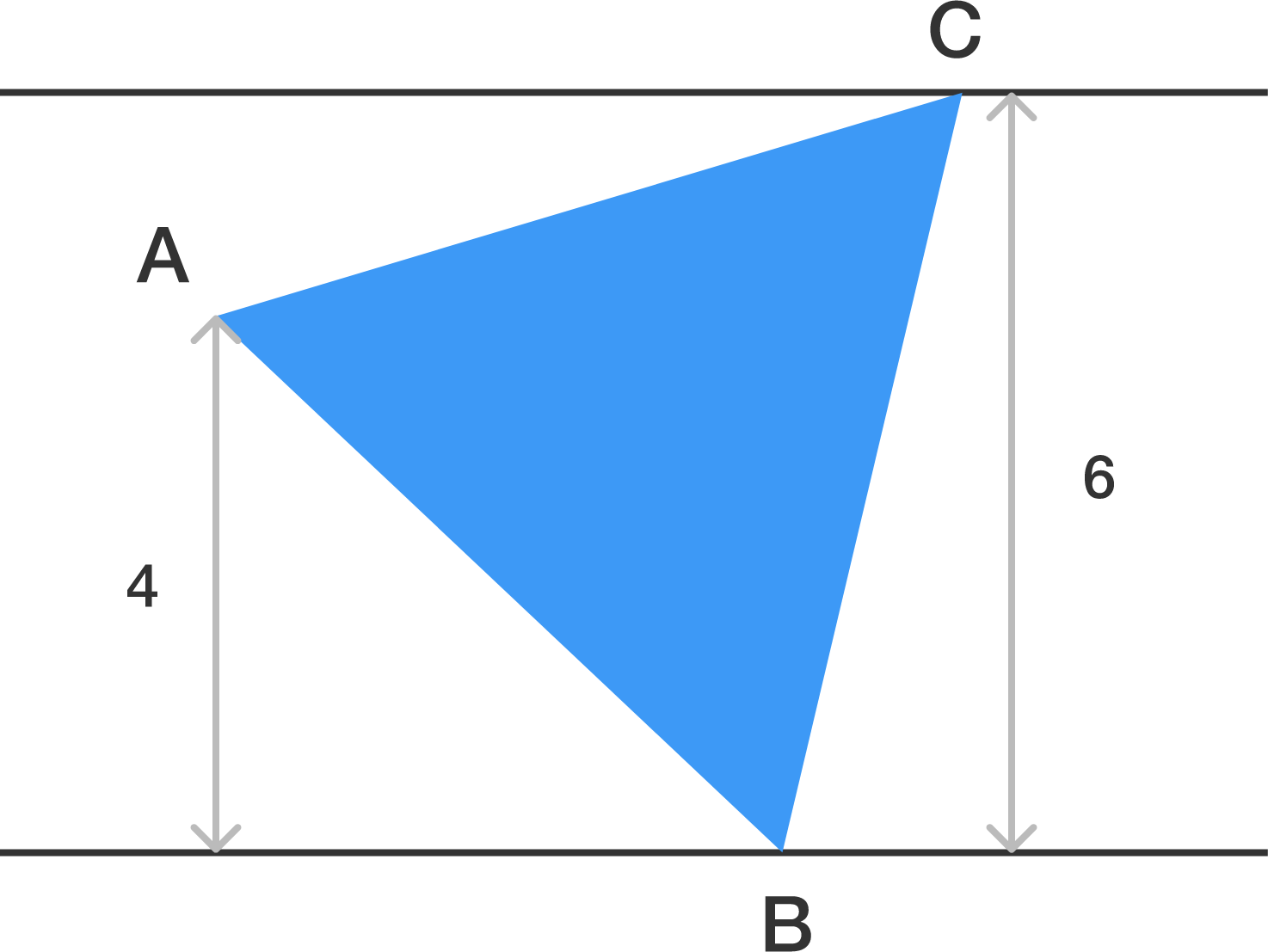Geometry

# 2D Coordinate Geometry: Level 4 Challenges

What is the area enclosed by $(y-x)^{2}=4$ and $(y+x)^{2}=4?$

The points $(3, 7), (6, 2),$ and $(2, k)$ are the vertices of a triangle. For how many real values of $k$ is the triangle a right triangle?

Tangents are drawn from $P (6,8)$ to the circle $x^2+y^2=r^2$. Find the radius of the circle such that the area of the triangle formed by tangents and chord of contact is maximum.Triangle $ABC$ has coodinates $A= (-4, 0)$, $B= (4 , 0)$, and $C= (0 , 3)$.

Let $P$ be the point in the first quadrant such that $\triangle ABP$ has half the area of $\triangle ABC$ but both triangles have the same perimeter.

What is the length of $CP?$ If your solution is in a form of $\sqrt{d}$, submit $d$ as the answer.

$ABC$ is an equilateral triangle such that vertices $B,C$ lie on two parallel lines at a distance of 6 units. If $A$ lies between the parallel lines at a distance 4 units from one of them, then the length of the side of the triangle is of the form $\large{A\sqrt{\frac{B}{C}}}$, where $A,B,C$ are co prime natural numbers.

Find $A+B+C$.×